# Scatter plots in R Language

• Last Updated : 10 Dec, 2021

A scatter plot is a set of dotted points to represent individual pieces of data in the horizontal and vertical axis. A graph in which the values of two variables are plotted along X-axis and Y-axis, the pattern of the resulting points reveals a correlation between them.

## R – Scatter plots

We can create a scatter plot in R Programming Language using the plot() function.

Syntax: plot(x, y, main, xlab, ylab, xlim, ylim, axes)

Parameters:

• x: This parameter sets the horizontal coordinates.
• y: This parameter sets the vertical coordinates.
• xlab: This parameter is the label for horizontal axis.
• ylab: This parameter is the label for vertical axis.
• main: This parameter main is the title of the chart.
• xlim: This parameter is used for plotting values of x.
• ylim: This parameter is used for plotting values of y.
• axes: This parameter indicates whether both axes should be drawn on the plot.

## Simple Scatterplot Chart

In order to create Scatterplot Chart:

1. We use the data set “mtcars”.
2. Use the columns “wt” and “mpg” in mtcars.

Example:

## R

 `input <- mtcars[, ``c``(``'wt'``, ``'mpg'``)]` `print``(``head``(input))`

Output: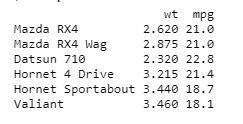## Creating a Scatterplot Graph

In order to create a Scatterplot graph:

1. We are using the required parameters to plot the graph.
2. In this ‘xlab’ describes the X-axis and ‘ylab’ describes the Y-axis.

Example:

## R

 `# Get the input values.` `input <- mtcars[, ``c``(``'wt'``, ``'mpg'``)]`   `# Plot the chart for cars with` `# weight between 1.5 to 4 and` `# mileage between 10 and 25.` `plot``(x = input\$wt, y = input\$mpg,` `    ``xlab = ``"Weight"``,` `    ``ylab = ``"Milage"``,` `    ``xlim = ``c``(1.5, 4),` `    ``ylim = ``c``(10, 25),        ` `    ``main = ``"Weight vs Milage"` `)`

Output:## Scatterplot Matrices

When we have two or more variables and we want to correlate between one variable and others so we use a scatterplot matrix.

pairs() function is used to create matrices of scatterplots.

Syntax: pairs(formula, data)

Parameters:

• formula: This parameter represents the series of variables used in pairs.
• data: This parameter represents the data set from which the variables will be taken.

Example:

## R

 `# Plot the matrices between` `# 4 variables giving 12 plots.`   `# One variable with 3 others` `# and total 4 variables.` `pairs``(~wt + mpg + disp + cyl, data = mtcars,` `    ``main = ``"Scatterplot Matrix"``)`

Output: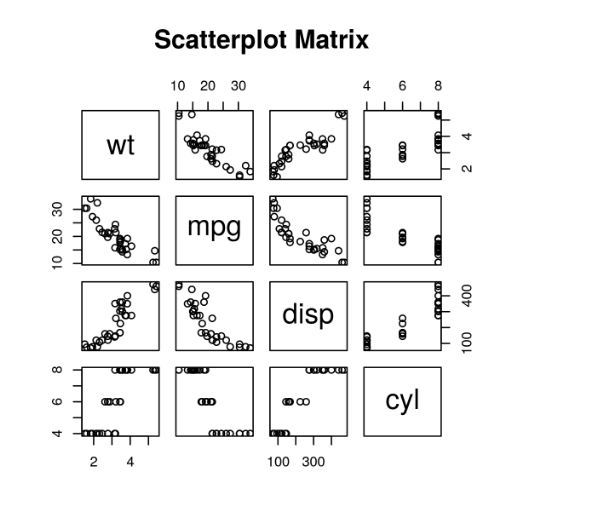## Scatterplot with fitted values

In order to create Scatterplot Chart:

1. We are using the ggplot2 package provides ggplot() and geom_point() function for creating a scatterplot.
2. Also we are using the columns “wt” and “mpg” in mtcars.

Example:

## R

 `# Loading ggplot2 package` `library``(ggplot2)` `    `  `# Creating scatterplot with fitted values.` `# An additional function stst_smooth` `# is used for linear regression.` `ggplot``(mtcars, ``aes``(x = ``log``(mpg), y = ``log``(drat))) +` `        ``geom_point``(``aes``(color = ``factor``(gear))) +` `        ``stat_smooth``(method = ``"lm"``,` `        ``col = ``"#C42126"``, se = ``FALSE``, size = 1` `)`

Output: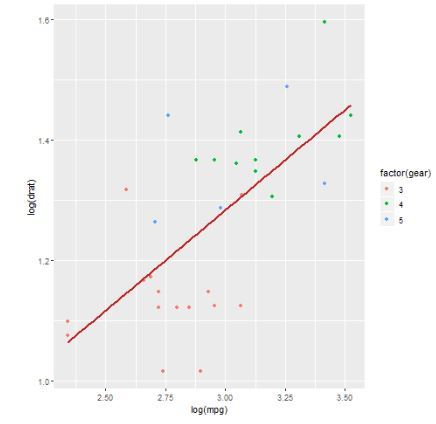## Adding title with dynamic name

To create Scatterplot Chart, Add a sub-title:

1. We use the additional function, In ggplot we add the data set “mtcars” with this adding ‘aes’, ‘geom_point’.
2. Use the Title, Caption, Subtitle.

Example:

## Python3

 `# Loading ggplot2 package` `library(ggplot2)` `    `  `# Creating scatterplot with fitted values.` `# An additional function stst_smooth` `# is used for linear regression.` `new_graph<``-``ggplot(mtcars, aes(x ``=` `log(mpg),` `                              ``y ``=` `log(drat))) ``+` `                    ``geom_point(aes(color ``=` `factor(gear))) ``+` `                    ``stat_smooth(method ``=` `"lm"``,` `                                ``col ``=` `"#C42126"``,` `                    ``se ``=` `FALSE, size ``=` `1``)`   `# in above example lm is used for linear regression` `# and se stands for standard error.` `# Adding title with dynamic name` `new_graph ``+` `labs(` `        ``title ``=` `"Relation between Mile per hours and drat"``,` `        ``subtitle ``=` `"Relationship break down by gear class"``,` `        ``caption ``=` `"Authors own computation"` `)`

Output: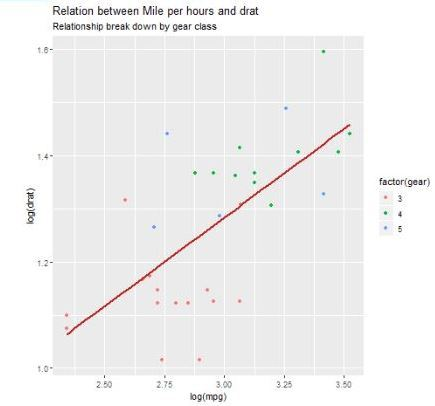## 3D Scatterplots

Here we will use scatterplot3D package to create 3D scatterplots, this package can plot scatterplot in 3D using scatterplot3d() methods.

## R

 `# 3D Scatterplot` `library``(scatterplot3d)` `attach``(mtcars)`   `scatterplot3d``(mpg, cyl, hp,` `              ``main = ``"3D Scatterplot"``)`

Output: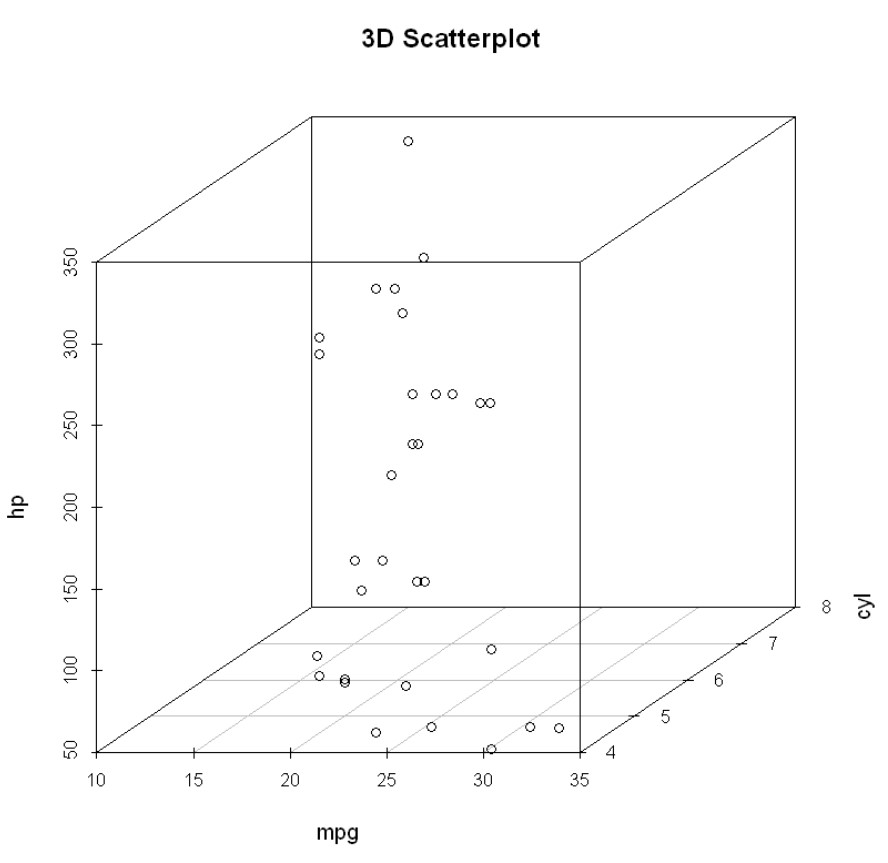My Personal Notes arrow_drop_up
Recommended Articles
Page :